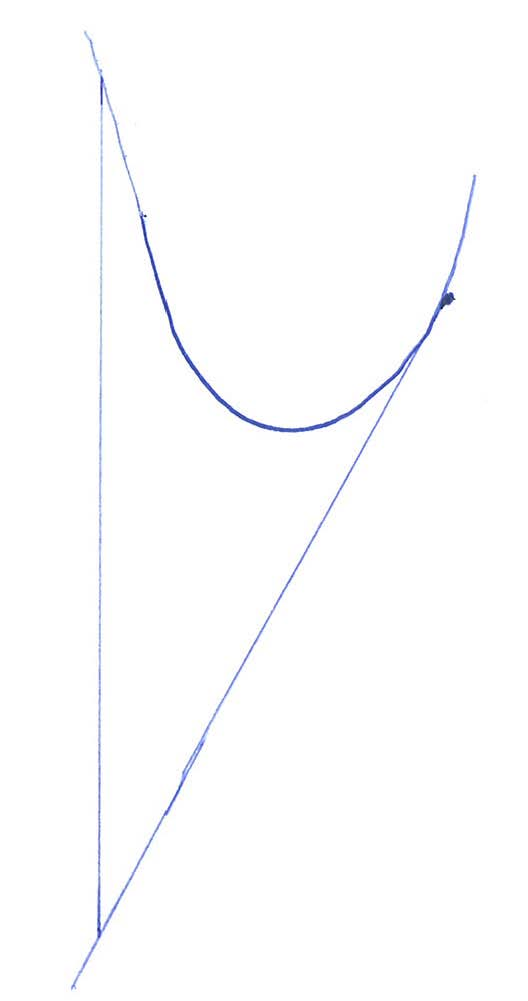http://zh.wikipedia.org/wiki/%E6%9C%80%E9%80%9F%E4%B8%8B%E9%99%8D%E6%B3%95
Gradient descent is based on the observation that if the multivariable functionis defined and differentiable in a neighborhood of a point, thendecreases fastest if one goes fromin the direction of the negative gradient ofatAndrew NG的coursera课程Machine learning的II. Linear Regression with One VariableGradient descent Intuition中的解释很好，比如在下图在右侧的点，则梯度是正数，是负数，即使当前的a减小http://blog.csdn.net/yudingjun0611/article/details/8147046

1. 梯度下降法

1）对于足够小的,  能保证在每一步都减小；
2）但是如果太小，梯度下降算法收敛的会很慢；

1）如果太小，就会收敛很慢；
2）如果太大，就不能保证每一次迭代都减小，也就不能保证收敛；

..., 0.001, 0.003, 0.01, 0.03, 0.1, 0.3, 1...

matlab源码：

2. theta0=0;
3. theta1=0;
4. t0=0;
5. t1=0;
6. while(1)
7.     for i=1:1:100 %100个点
8.         t0=t0+(theta0+theta1*X(i,1)-Y(i,1))*1;
9.         t1=t1+(theta0+theta1*X(i,1)-Y(i,1))*X(i,1);
10.     end
11.     old_theta0=theta0;
12.     old_theta1=theta1;
13.     theta0=theta0-0.000001*t0 %0.000001表示学习率
14.     theta1=theta1-0.000001*t1
15.     t0=0;
16.     t1=0;
17.     if(sqrt((old_theta0-theta0)^2+(old_theta1-theta1)^2)<0.000001) % 这里是判断收敛的条件，当然可以有其他方法来做
18.         break;
19.     end
20. end

2. 随机梯度下降法

matlab源码：